CBSE Class 10 Sample Paper for 2022 Boards - Maths Basic [MCQ]

Class 10
Solutions of Sample Papers for Class 10 Boards

## Polynomials are everywhere. They play a key role in the study of algebra, in analysis and on the whole many mathematical problems involving them. Since, polynomials are used to describe curves of various types engineers use polynomials to graph the curves of roller coasters.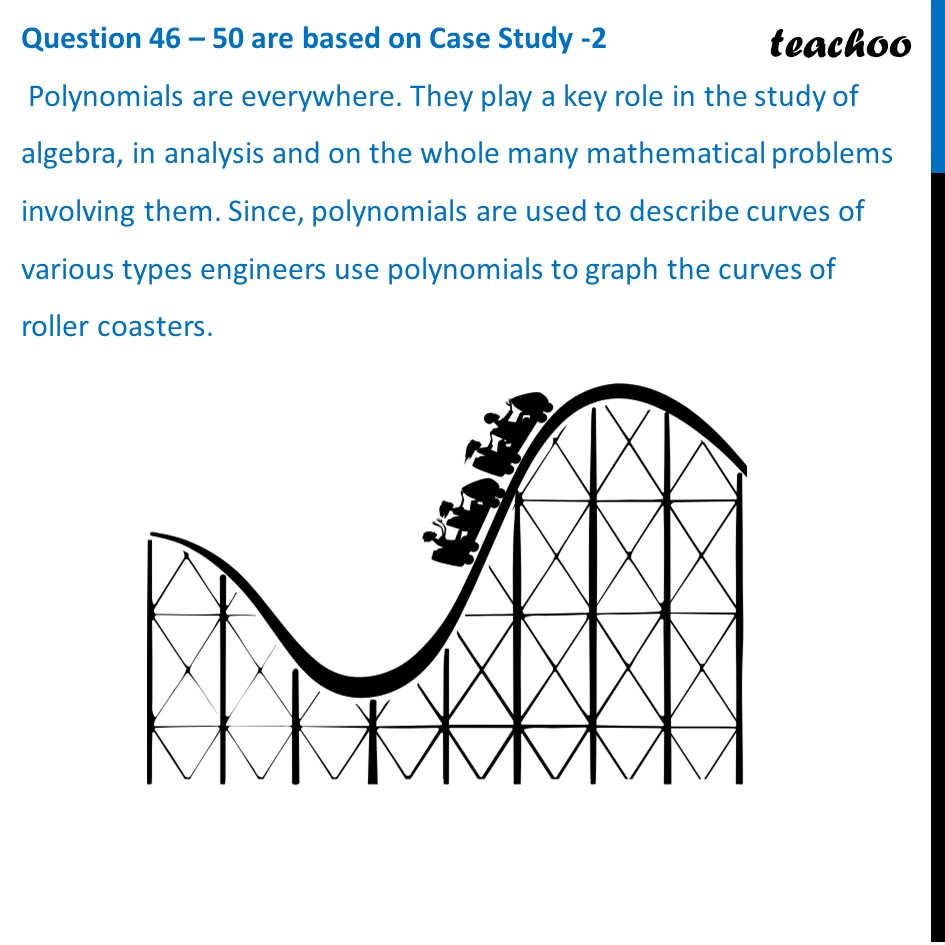###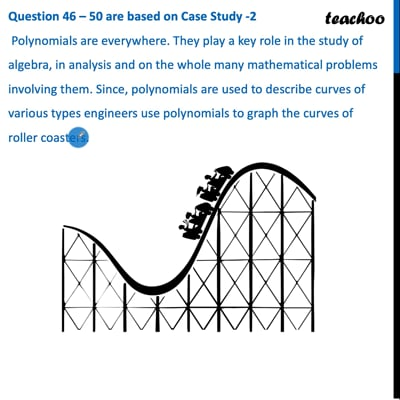This video is only available for Teachoo black users If the Roller Coaster is represented by the following graph y = p(x) , then name the type of the polynomial it traces. (a) Linear           (b) Quadratic      (c) Cubic   (d) Bi-quadratic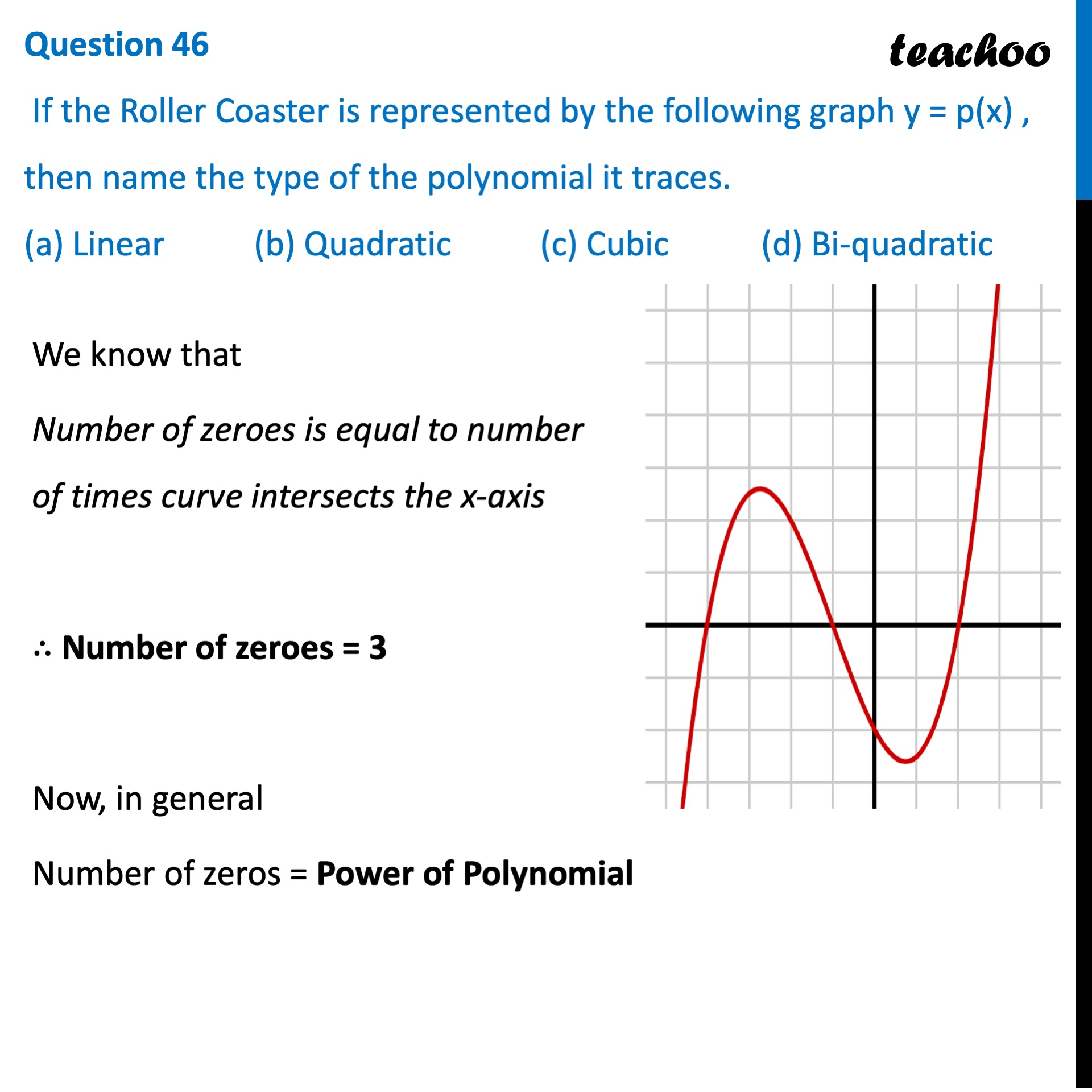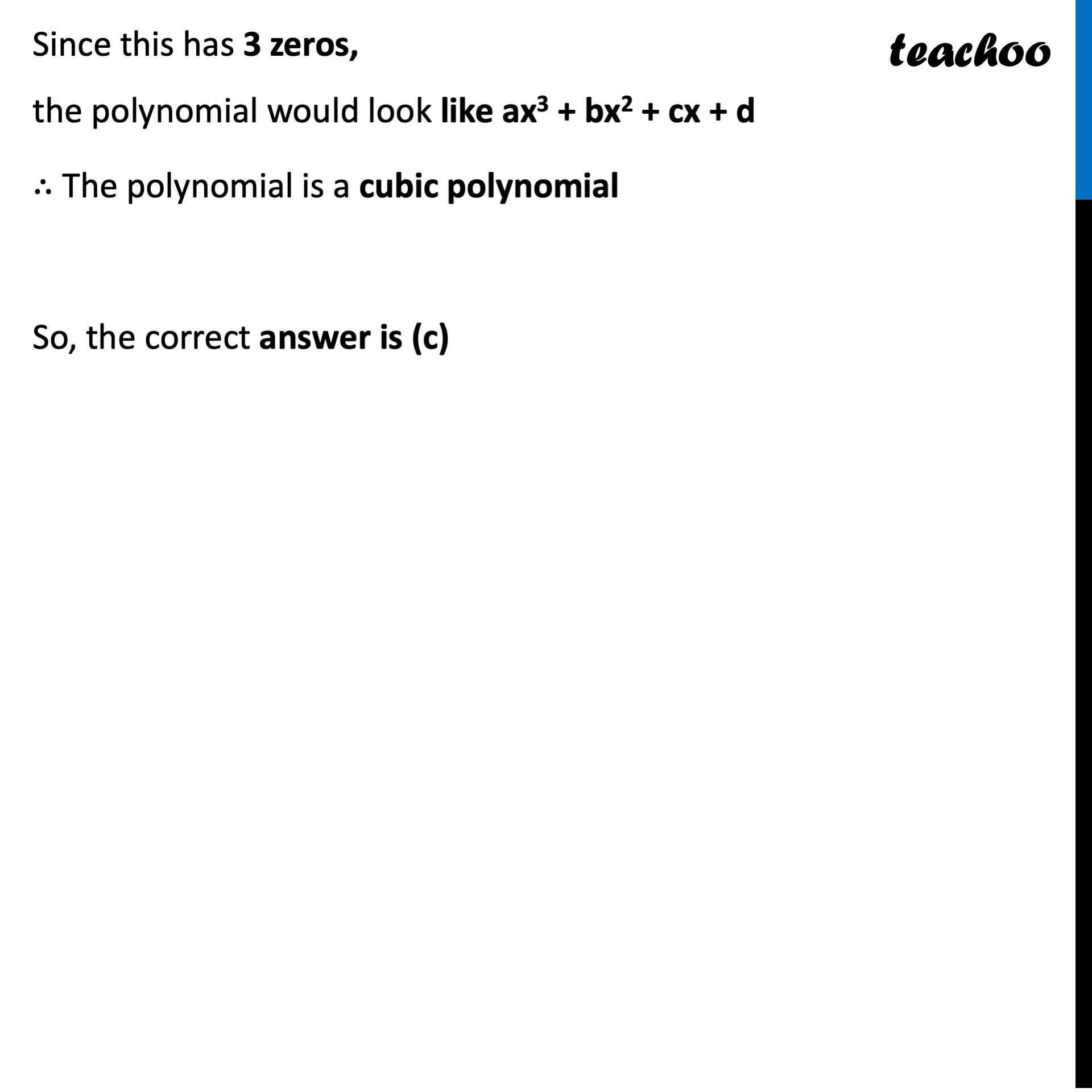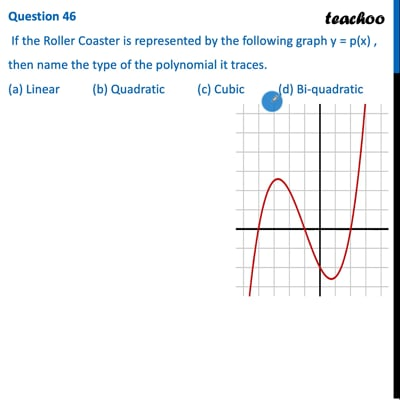This video is only available for Teachoo black users

Learn in your speed, with individual attention - Teachoo Maths 1-on-1 Class

### Transcript

Question 46 – 50 are based on Case Study -2 Polynomials are everywhere. They play a key role in the study of algebra, in analysis and on the whole many mathematical problems involving them. Since, polynomials are used to describe curves of various types engineers use polynomials to graph the curves of roller coasters. Question 46 If the Roller Coaster is represented by the following graph y = p(x) , then name the type of the polynomial it traces. (a) Linear (b) Quadratic (c) Cubic (d) Bi-quadratic We know that Number of zeroes is equal to number of times curve intersects the x-axis ∴ Number of zeroes = 3 Now, in general Number of zeros = Power of Polynomial Since this has 3 zeros, the polynomial would look like ax3 + bx2 + cx + d ∴ The polynomial is a cubic polynomial So, the correct answer is (c)